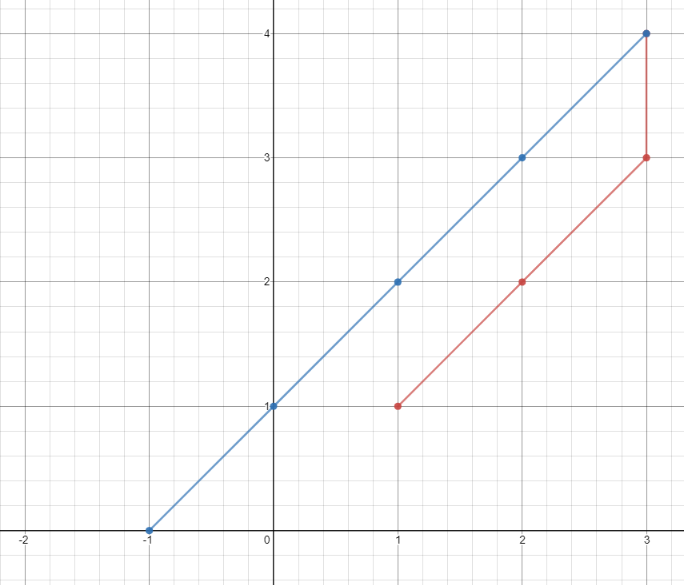## Algorithm

Problem Name: 1266. Minimum Time Visiting All Points

On a 2D plane, there are `n` points with integer coordinates `points[i] = [xi, yi]`. Return the minimum time in seconds to visit all the points in the order given by `points`.

You can move according to these rules:

• In `1` second, you can either:
• move vertically by one unit,
• move horizontally by one unit, or
• move diagonally `sqrt(2)` units (in other words, move one unit vertically then one unit horizontally in `1` second).
• You have to visit the points in the same order as they appear in the array.
• You are allowed to pass through points that appear later in the order, but these do not count as visits.

Example 1:```Input: points = [[1,1],[3,4],[-1,0]]
Output: 7
Explanation: One optimal path is [1,1] -> [2,2] -> [3,3] -> [3,4] -> [2,3] -> [1,2] -> [0,1] -> [-1,0]
Time from [1,1] to [3,4] = 3 seconds
Time from [3,4] to [-1,0] = 4 seconds
Total time = 7 seconds```

Example 2:

```Input: points = [[3,2],[-2,2]]
Output: 5
```

Constraints:

• `points.length == n`
• `1 <= n <= 100`
• `points[i].length == 2`
• `-1000 <= points[i], points[i] <= 1000`

## Code Examples

### #1 Code Example with Java Programming

```Code - Java Programming```

``````
class Solution {
public int minTimeToVisitAllPoints(int[][] points) {
int time = 0;
for (int i = 1; i < points.length; i++) {
int[] prevPoint = points[i - 1];
int[] currPoint = points[i];
time += Math.max(
Math.abs(prevPoint - currPoint), Math.abs(prevPoint - currPoint)
);
}
return time;
}
}
``````
Copy The Code &

Input

cmd
points = [[1,1],[3,4],[-1,0]]

Output

cmd
7

### #2 Code Example with Javascript Programming

```Code - Javascript Programming```

``````
const minTimeToVisitAllPoints = function(points) {
let res = 0
for(let i = 1; i < points.length; i++) {
res += calc(points[i], points[i - 1])
}
return res

function calc(p1, p2) {
const [x1, y1] = p1,  [x2, y2] = p2
const { abs, min } = Math
const deltaX = abs(x1 - x2), deltaY = abs(y1 - y2)

return min(deltaX, deltaY) + abs(deltaX - deltaY)
}
};
``````
Copy The Code &

Input

cmd
points = [[1,1],[3,4],[-1,0]]

Output

cmd
7

### #3 Code Example with C# Programming

```Code - C# Programming```

``````
using System;

namespace LeetCode
{
public class _1266_MinimumTimeVisitingAllPoints
{
public int MinTimeToVisitAllPoints(int[][] points)
{
var result = 0;
for (int i = 1; i < points.Length; i++)
result += Math.Max(
Math.Abs(points[i] - points[i - 1]),
Math.Abs(points[i] - points[i - 1]));

return result;
}
}
}
``````
Copy The Code &

Input

cmd
points = [[1,1],[3,4],[-1,0]]

Output

cmd
7